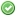rfc:pow-operator

This is an old revision of the document!

# PHP RFC: Power Operator

## Introduction

This proposal is three-fold:

1. Turn the existing pow() function into a language construct.
2. Introduce an exponential (right associative) operator `**`.
• Easier to read and shorter to write.
• Can be found in other languages.
3. Introduce an exponential assignment operator `**=`

## Proposal

pow() as language construct

No changes to existing code, safe for one:

`var_dump(pow([], 2)); // int(0) instead of float(0)`

Using `pow()` as a callback will still work as expected:

`call_user_func('pow', 2, 3); // 8`

Power operator

A short working example:

`echo 2 ** 3; // 8`

```\$base = gmp_init(2);
\$exponent = 3;
var_dump(\$base ** \$exponent);

// output
object(GMP)#3 (1) {
["num"]=>
string(1) "8"
}```

Example of exponent assignment:

```\$x = 2;
\$x **= 3;
echo \$x; // 8```

Important

The proposed associativity is right, just like how power towers work.

The operator precedence is:

• higher than the bitwise not (~) and unary minus,
• lower than array dereferencing.

Examples:

```echo 2 ** 3 ** 2; // 512 (not 64)
echo -3 ** 2; // -9 (not 9)
echo 1 - 3 ** 2; // -8
echo ~3 ** 2; // -10 (not 16)```

## Discussion

Should `-2 ** 3` evaluate to `9` instead of `-9`?

According to the following resources, the scale tips more towards having the exponent precede the unary minus:

Similar languages

• D
• F#
• Fortran
• Freemat
• Mathematica / Matlab / Scilab
• Octave
• Perl
• Python
• R
• Ruby
• Sage
• VB / Basic

Dissimilar languages

• Bash
• Cobol
• ColdFusion
• Excel
• Tcl

Should `2 ** 3 ** 2` yield `64` (left associative), `512` (right associative) or throw an error (non associative)?

The exponent operator evaluation order should be based on Tetration and therefore be right associative.

Languages with left associative exponential operator

• VB (not by choice imho)
• Basic
• Octave
• Matlab
• ColdFusion

Languages with right associative exponential operator

• D
• R
• F#
• Ruby
• Perl
• Python
• Mathematica
• Freemat
• Scilab
• Tcl (changed from left associative!)
• Cobol
• Fortran
• Sage
• Bash

Languages with non associative exponential operator

Sources

PHP 5.6

## Impact to Existing Extensions

The opcode `ZEND_POW <165>` and `ZEND_ASSIGN_POW <166>` is added.

External extensions such as vld or phpdbg would have to be updated, but I'm not aware of any core extensions that would otherwise be affected.

None.

## Vote

Voting will be based on the following:

• Turn `pow()` into a language construct,
• Add the power operators `**` and `**=`,
• Add `ZEND_POW` and `ZEND_ASSIGN_POW` opcodes.

Changes from 0.1:

• A option is added to vote for a non-associative `**` operator. See also: Discussion. This counts as an inclusion vote.

A two third majority is required for acceptance.

Should PHP get a power operator in 5.6?
Real name Yes, right associative Yes, non associative No
aharvey (aharvey)ajf (ajf)auroraeosrose (auroraeosrose)bmajdak (bmajdak)bwoebi (bwoebi)chobieeee (chobieeee)cyberspice (cyberspice)datibbaw (datibbaw)daverandom (daverandom)davey (davey)derick (derick)dm (dm)googleguy (googleguy)guilhermeblanco (guilhermeblanco)kassner (kassner)krakjoe (krakjoe)kriscraig (kriscraig)levim (levim)lstrojny (lstrojny)mbeccati (mbeccati)mfonda (mfonda)nikic (nikic)peehaa (peehaa)philstu (philstu)pollita (pollita)rasmus (rasmus)rdlowrey (rdlowrey)salathe (salathe)seld (seld)stas (stas)uw (uw)willfitch (willfitch)Count: 22 1 9

Voting ends on 5th of January 2014.

## Patches and Tests